# SBI PO Prelims Quantitative Aptitude Quiz- 42

## SBI PO Prelims Quantitative Aptitude Quiz

Quantitative Aptitude is a very essential and scoring section of almost every competitive exam. Candidates appear to be having difficulty with Quantitative Aptitude Questions, so they need to practice more. To help you prepare better, we are providing you with the SBI PO Prelims Quantitative Aptitude Quiz. This SBI PO Prelims Quantitative Aptitude Quiz is based on the latest and updated pattern. Candidates will be furnished with a detailed explanation for each question in this SBI PO Prelims Quantitative Aptitude Quiz. This SBI PO Prelims Quantitative Aptitude Quiz includes all types of questions that are generally asked in exams. This SBI PO Prelims Quantitative Aptitude Quiz is absolutely free of cost. This SBI PO Prelims Quantitative Aptitude Quiz will assist aspirants in achieving a good score in their upcoming examination.

1. Shikha calculates his Profit % on SP whereas Ramesh calculates profit percent on CP. They find that the difference in their profit is Rs. 1000. If SP of Both are same and both gets 25% profit. Find their SP.
(a)Rs. 5000
(b)Rs. 4500
(c)Rs. 3000
(d) Rs. 2500
(e) None of the above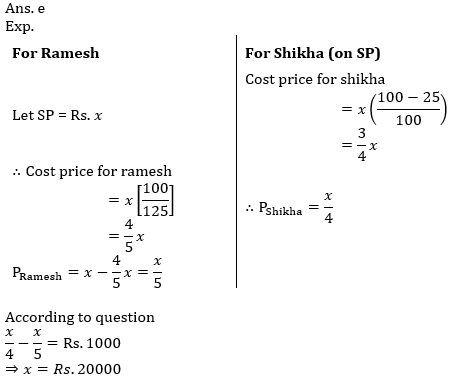2. In a company, there are 75% skilled workers and remaining ones are unskilled. 80% of skilled workers and 20% of unskilled workers are permanent. If number of temporary workers is 126, then what is the number of total workers?
(a) 480
(b) 510
(c) 360
(d) 377
(e) None of these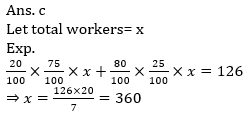3. Prema goes to a shop to buy a sofa set costing Rs. 13,080. The rate of sales tax is 9%. She tells the shopkeeper to reduce the price of the sofa set to such an extent that she has to pay Rs. 13080 inclusive of sales tax. Find the percentage reduction needed in the price of the sofa set to just satisfy her requirement.
(a) 8.33%
(b) 8.26%
(c) 9%
(d) 8.5%
(e) None of these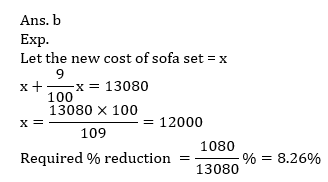4. Jonny has two cycles and one rickshaw. The rickshaw is worth Rs. 96. If he sells the rickshaw along with the first cycle, he has an amount double that of the value of the second cycle. But if he decides to sell the rickshaw along with the second cycle, the amount received would be less than the value of first cycle by Rs. 306. What is the value of first cycle?
(a) Rs. 900
(b) Rs. 600
(c) Rs. 498
(d) Rs. 520
(e) None of these5. A mother divided an amount of Rs. 61,000 between her two daughters aged 18 years and 16 years respectively and deposited their shares in a bond. If the interest rate is 20% compounded annually and if each received the same amount as the other when they attained the age of 20 years, their shares are
(a) Rs. 35,600 and Rs. 25,400
(b) Rs. 30500 each
(c) Rs. 24,000 and Rs. 37000
(d) Rs. 36,000 and Rs 25,000
(e) None of these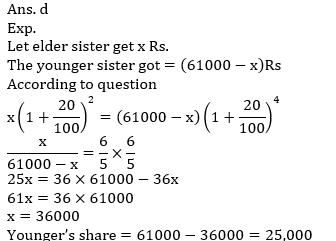Directions (6-10): In each of these questions two equations (I) and (II) are given you have to solve both the equations and give answer.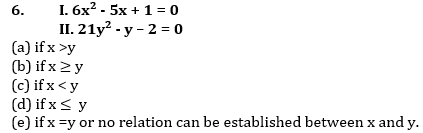.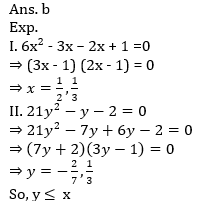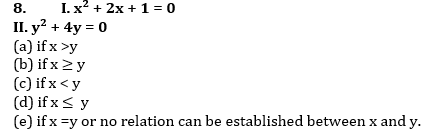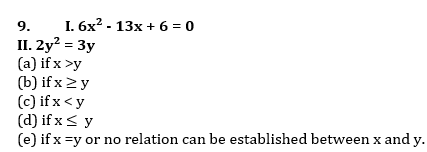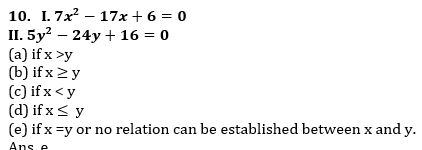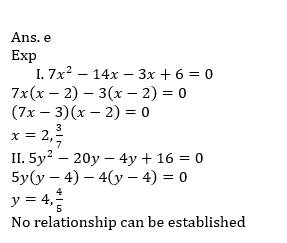###Click to Buy Bank MahaCombo Package

Recommended PDF’s for:

#### Most important PDF’s for Bank, SSC, Railway and Other Government Exam : Download PDF Now

AATMA-NIRBHAR Series- Static GK/Awareness Practice Ebook PDF Get PDF here
The Banking Awareness 500 MCQs E-book| Bilingual (Hindi + English) Get PDF here
AATMA-NIRBHAR Series- Banking Awareness Practice Ebook PDF Get PDF here
Computer Awareness Capsule 2.O Get PDF here
AATMA-NIRBHAR Series Quantitative Aptitude Topic-Wise PDF Get PDF here
AATMA-NIRBHAR Series Reasoning Topic-Wise PDF Get PDF Here
Memory Based Puzzle E-book | 2016-19 Exams Covered Get PDF here
Caselet Data Interpretation 200 Questions Get PDF here
Puzzle & Seating Arrangement E-Book for BANK PO MAINS (Vol-1) Get PDF here
ARITHMETIC DATA INTERPRETATION 2.O E-book Get PDF here

3